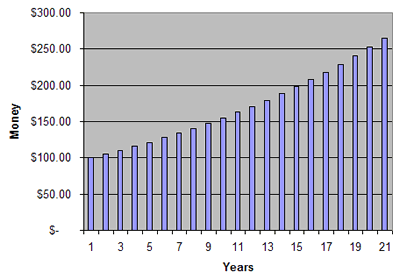# How does compound interest work?

The term "compound interest" refers to the growth of money over time. Typically, it is used when money is invested in a bank. Over time, the bank pays interest as a sort of "rent" for borrowing your money. If you keep that interest in the bank too, eventually it will earn its own interest.

Here is a graph showing what happens to $100 invested at 5% interest for 20 years:As you can see, the money earns more and more each year. In the first year the bank pays 5% interest, which equals$100 multiplied by 1.05. The next year they pay you 5% interest on the $105 you now have in the bank, so your balance increases even more to$110.25. After 20 years, the money has turned into \$265.33.

To determine the future value of money put in the bank, use the future value formula:

$$F = P*(1+r)^n$$

F is the future value, or how much your money will be worth in the future. P is the present value, or how much you have saved right now. r is the interest rate, expressed as a decimal (.05 for 5%, .12 for 12% etc). n is the number of years the money will earn interest.

Banks typically don't pay you all the interest at the end of the year, like in the example above. Instead, many pay monthly. In that case, divide the interest rate (r) by 12, but multiply the number of years (n) by 12 to convert to months. But, do banks pay you based on how much you have in your account at the end of each month? No. Instead many use the average daily balance and then pay you once per month.

It is common to use calculate interest with what's called continuous interest. In this situation, the bank is paying (or charging, for a loan) interest constantly, but at an incredibly low rate each time. The formula for this is:

$$F = Pe^{rt}$$

Here, the future value F equals the present value P times e(Euler's Number) raised to the (rate * time) exponential. It gives a similar value to the one returned with the simple interest formula we showed first.